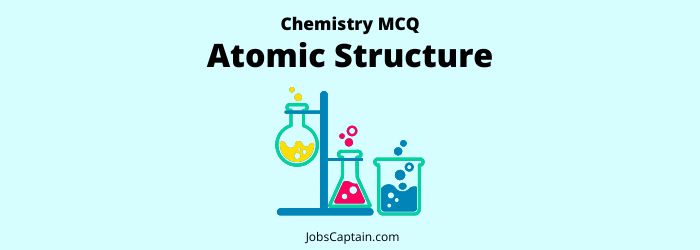# Atomic Structure MCQ Quiz: ChemistryWelcome to our Chemistry Atomic Structure MCQ Quiz! In this quiz, we’ll test your knowledge on one of the most important topics in chemistry – atomic structure. From the basics of atoms and their structure to more complex topics like the electronic configuration of atoms, we’ll be covering everything you need to know to ace this quiz!

Question 1. Which of the following is not a part of an atom?

(A) Proton

(B) Neutron

(C) Photon

(D) Electron

(C) Photon

Question 2. A single type of atom is found in _______.

(A)Native elements

(B) Mixture of minerals

(C)Compounds of minerals

(D) None of the above

(A)Native elements

Question 3. Constituents of atomic nucleus are _________.

(A) Proton and neutron

(B) Electron and proton

(C) Electron and neutron

(D) Proton, neutron and electron

(A) Proton and neutron

Question 4.  Which of the following statement about molecular structure is correct?

(A) Proton, neutron and electron all are inside the nucleus

(B) Proton and neutron are inside the nucleus and electrons revolve around the nucleus

(C) Electron and proton are inside the nucleus and neutron revolve around the nucleus

(D) Neutron and electron are found inside the nucleus and protons revolves around the nucleus

(B) Proton and neutron are inside the nucleus and electrons revolve around the nucleus

Question 5. The atomic nucleus was discovered by _________.

(A) Thomson

(B) Einstein

(C) Dalton

(D) Rutherford

(D) Rutherford

Question 6. Radioactivity was discovered by ________.

(B) Bohr

(C) Becquerel

(D) Rutherford

(C) Becquerel

Question 7. Isotopes are those atoms of the same element which have _________.

(A) None of the above

(B) Atomic number and atoms mass both are same

(C) Atomic mass is different but atomic number is same

(D) Atomic mass is same but atomic number is different

(C) Atomic mass is different but atomic number is same

Question 8. Isotopes is that nuclei of atomic nucleus in which?

(A) Number of both protons and neutrons is different

(B) Number of both protons and neutrons is same

(C) Number of protons is same but number of neutrons is different

(D) Number of neutrons is same but number of protons is different

(C) Number of protons is same but number of neutrons is different

Question 9. The isoneutronic group, in the following is ________.

(A) 6C14, 7N15, 8O16

(B) 6C14, 7N14, 8O16

(C) 6C12, 7N14, 8N18

(D) 6C12, 7N14, 8O16

(A) 6C14, 7N15, 8O16

Question 10. Radioactivity is measured by _________.

(A)  Ammeter

(B) Seismometer

(C) Geiger Counter

(D) Hydrometer

(C) Geiger Counter

Question 11. Match List-I with List-II and select the correct answer using the code given below the lists.

 List-I (Characteristic) List-II (Particle) (a) Zero mass 1. Positron (b) Fractional charge 2. Neutrino (c) Fractional spin 3. Quark (d) Integral spin 4. Photon

Code

 (a) (b) (c) (d) (A) 3 2 1 4 (B) 2 3 4 1 (C) 3 2 4 1 (D) 2 3 1 4
(D) 2 3 1 4

Question 12. The atoms, in which the number of protons is same but the number of neutrons is different, are known asf ?

(A) Isotopes

(B) Isotones

(C) Isomers

(D) Isobars

(A) Isotopes

Question 13. What is the mass number of an element, the atom of which contains two protons, two neutrons and two electrons?

(A) 8

(B) 6

(C) 4

(D) 2

(C) 4

Question 14. Regarding the atom of a chemical element, the magnetic quantum number refers to?

(A) Spin

(B) Size

(C) Shape

(D) Orientation

(D) Orientation

Question 15. In an atom, the order of filling up of the orbitals is governed by?

(A) Pauli’s exclusion principle

(B) Hund’s rule

(C) Heisenberg’s uncertainty principle

(D) Aufbau’s principle

(D) Aufbau’s principle

Question 16. Which of the following carries a negative charge?

(A) Gamma rays

(B) Beta particles

(C) Alpha particles

(D) X-rays

(B) Beta particles

Question 17. The Nucleus of Helium has?

(A) One proton and two neutrons

(B) Two protons and two neutrons

(C) Two protons

(D) Only one neutron

(B) Two protons and two neutrons

Question 18. The alpha particle carries two positive charges. Its mass is very nearly equal to that of?

(A) Two positrons are each positron carries a single positive charge

(B) Sum of masses of two positrons and two neutrons

(C) An atom of helium

(D) Two protons

(C) An atom of helium

Question 19. Which one of the following pairs constitutes particle-antiparticle pair?

(A) Neutron-Neutrino

(B) Photon-Electron

(C) Proton-Neutron

(D) Electron-Positron

(D) Electron-Positron

Question 20. Which of the following is not a subatomic particle?

(A) Electron

(B) Deuteron

(C) Proton

(D) Neutron

(B) Deuteron

Question 21. In atom, neutron was discovered by?

(A) Newton

(B) Rutherford